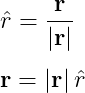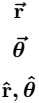# How to denote vector bold(a = |a| â) in LaTeX?

You may have seen in various books or science documents that vectors are written in bold form without arrow marks.

For this, you need to know how to convert a text’s font weight from normal to bold. And this concept will be discussed in this tutorial. So, let’s learn something interesting …

## Use \textbf command for vector bold in LaTeX

In latex, font weight of text is converted from normal to bold with the help of \textbf command. And this is the default command. For this, you do not need to use any package.

\documentclass{article}
\begin{document}
$\hat{r} = \frac{\textbf{r}}{\mid\textbf{r}\mid}$
$\textbf{r} = \mid\textbf{r}\mid\hat{r}$
\end{document}

Output :And you can use this command in both text and math mode.

However, font-style italics with font-weight bold are used when denoting vectors in different documents.

\documentclass{article}
\begin{document}
$\textbf{a b c}$
$\textbf{\textit{a b c}}$
$\textbf{\textit{p q r}}$
\end{document}

Output :## Use physics package for vector bold symbol

The physics package contains the \vectorbold command for vector bold. And this \vectorbold command is written in short form as \vb.

\documentclass{article}
\usepackage{physics,amsmath}
\begin{document}
$\vectorbold{(p \times q)\cdot r}$
$\vb{p \cdot (q \times r)}$
\end{document}

Output :However, if you add a star symbol with the \vb command, the text font style will be converted to italic. For example

\documentclass{article}
\usepackage{physics,amsmath}
\begin{document}
$\vectorbold*{(p \times q)\cdot r}$
$\vb*{p \cdot (q \times r)}$
\end{document}

Output :## Use arrow symbol with vector bold in LaTeX

In many cases, arrow and font-weight bold are used simultaneously on vector variables.

\documentclass{article}
\usepackage{physics}
\begin{document}
$\vectorbold*{\va{r}}$
$\vb*{\va{\theta}}$
$\vb*{\vu{r},\vb{\theta}}$
\end{document}

Output :#### Md Jidan Mondal

LaTeX expert with over 10 years of experience in document preparation and typesetting. Specializes in creating professional documents, reports, and presentations using LaTeX.